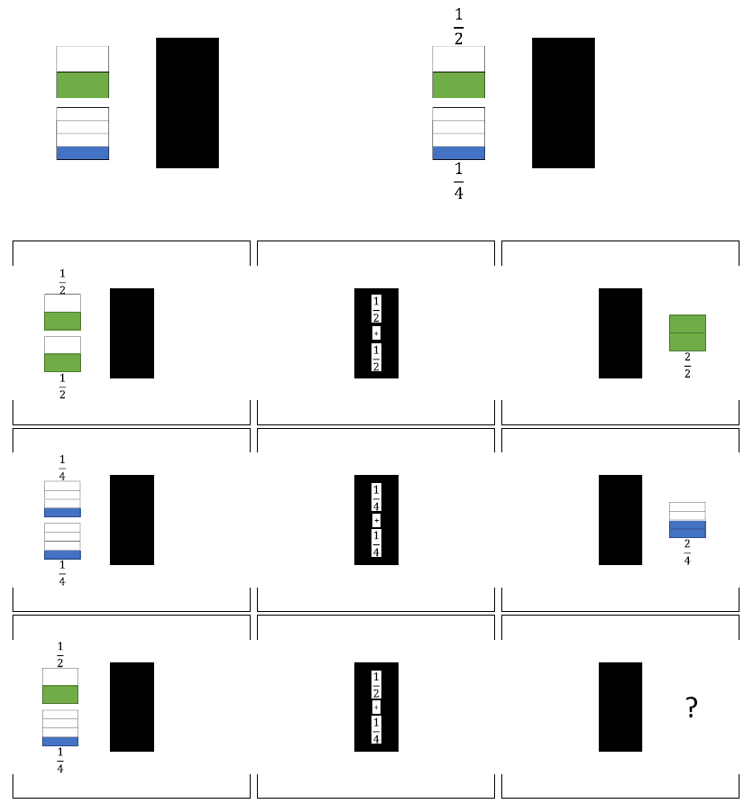Match Fishtank is now Fishtank Learning!

# Fraction Operations

## Objective

Add two fractions where one denominator is a divisor of the other using the denominators 2, 3, 4, 5, 6, 8, 10, and 12.

## Common Core Standards

### Core Standards

?

• 4.NF.B.3.A — Understand addition and subtraction of fractions as joining and separating parts referring to the same whole.

• 4.NF.B.3.B — Decompose a fraction into a sum of fractions with the same denominator in more than one way, recording each decomposition by an equation. Justify decompositions, e.g., by using a visual fraction model. Examples: 3/8 = 1/8 + 1/8 + 1/8 ; 3/8 = 1/8 + 2/8 ; 2 1/8 = 1 + 1 + 1/8 = 8/8 + 8/8 + 1/8.

?

• 4.NF.A.1

## Criteria for Success

?

1. Make sense of a three-act task and persevere in solving it (MP.1).
2. Add fractions where one denominator is a divisor of the other and where the sum is between 1 and 2, by replacing one fraction with an equivalent fraction in such a way as to produce an equivalent sum of fractions with like denominators.
3. (Optional) Simplify solutions by rewriting them using the largest possible unit.

## Tips for Teachers

?

• Lesson 7 goes beyond the explicit expectations of 4.NF.3. However, students will add fractions with denominators of 10 and 100 in Unit 7 (4.NF.5). Thus, today’s lesson is intended to prepare students for that work in Unit 7. The Progressions note that “students might encounter [such] situations, for example using a strip of paper or a tape diagram to reason that $\frac{1}{3}+\frac{1}{6}=\frac{2}{6}+\frac{1}{6}=\frac{3}{6}=\frac{1}{2}$” (NF Progression, p. 16).
• In place of the Problem Set, you might decide to play the following NCTM Illuminations game, Fraction Game. This game can also be converted to a paper version if you don’t want to use the computer version.

#### Fishtank Plus

• Problem Set
• Student Handout Editor
• Vocabulary Package

?

### Problem 1

Act 1: Watch the following video: Black box - Act 1.

What will the sum of $\frac{1}{2} + \frac{1}{4}$ be?

#### References

101Questions Black Box 2

Black Box 2 by is made available on 101Questions under the CC BY 3.0 license. Accessed July 17, 2018, 1:45 p.m..

Modified by Fishtank Learning, Inc.
YouTube Video: “Black box - Act 1” by msbritton215

Black box - Act 1 by msbritton215 is made available by YouTube under the Standard YouTube License. Accessed Oct. 23, 2019, 5:27 p.m..

### Problem 2

Act 2: Use the following information to solve.#### References

101Questions Black Box 2

Black Box 2 by is made available on 101Questions under the CC BY 3.0 license. Accessed July 17, 2018, 1:45 p.m..

Modified by Fishtank Learning, Inc.

### Problem 3

Act 3: Reveal the answer: Black box - Act 3.

#### References

101Questions Black Box 2

Black Box 2 by is made available on 101Questions under the CC BY 3.0 license. Accessed July 17, 2018, 1:45 p.m..

Modified by Fishtank Learning, Inc.
YouTube Video: “Black box - Act 3” by msbritton215

Black box - Act 3 by msbritton215 is made available by YouTube under the Standard YouTube License. Accessed Oct. 23, 2019, 5:27 p.m..

### Problem 4

Solve. Show or explain your work.

a.   ${{1\over2}+{1\over8}}$

b.   ${{1\over5}+{3\over10}}$

c.   ${{2\over3}+{1\over6}}$

d.   ${{3\over4}+{5\over12}}$

#### References

EngageNY Mathematics Grade 4 Mathematics > Module 5 > Topic D > Lesson 21Concept Development

Grade 4 Mathematics > Module 5 > Topic D > Lesson 21 of the New York State Common Core Mathematics Curriculum from EngageNY and Great Minds. © 2015 Great Minds. Licensed by EngageNY of the New York State Education Department under the CC BY-NC-SA 3.0 US license. Accessed Dec. 2, 2016, 5:15 p.m..

Modified by Fishtank Learning, Inc.
EngageNY Mathematics Grade 4 Mathematics > Module 5 > Topic D > Lesson 20Concept Development

Grade 4 Mathematics > Module 5 > Topic D > Lesson 20 of the New York State Common Core Mathematics Curriculum from EngageNY and Great Minds. © 2015 Great Minds. Licensed by EngageNY of the New York State Education Department under the CC BY-NC-SA 3.0 US license. Accessed Dec. 2, 2016, 5:15 p.m..

Modified by Fishtank Learning, Inc.

## Problem Set & Homework

#### Discussion of Problem Set

• How did you determine which amounts could have been what Jake added to the jug in #1?
• In #2c, did you use sixths as the common denominator? Explain how thirds could be used as the common denominator.
• For #5, what strategies did you use to estimate if the sum would be between 0 and 1 or 1 and 2?
• Why is it important to have common denominators when adding fractions?
• How can you find the sum of two fractions without using a model?
• What benefit can you see in expressing a fraction as a mixed number or a mixed number as a fraction?

?

Solve. Show or explain your work.

1.  ${{5\over8}+{2\over4}=}$ _____

2.  ${{2\over3}+{7\over12}=}$ _____

#### References

EngageNY Mathematics Grade 4 Mathematics > Module 5 > Topic D > Lesson 20Exit Ticket, Question #1

Grade 4 Mathematics > Module 5 > Topic D > Lesson 20 of the New York State Common Core Mathematics Curriculum from EngageNY and Great Minds. © 2015 Great Minds. Licensed by EngageNY of the New York State Education Department under the CC BY-NC-SA 3.0 US license. Accessed Dec. 2, 2016, 5:15 p.m..

Modified by Fishtank Learning, Inc.
EngageNY Mathematics Grade 4 Mathematics > Module 5 > Topic D > Lesson 21Exit Ticket, Question #2

Grade 4 Mathematics > Module 5 > Topic D > Lesson 21 of the New York State Common Core Mathematics Curriculum from EngageNY and Great Minds. © 2015 Great Minds. Licensed by EngageNY of the New York State Education Department under the CC BY-NC-SA 3.0 US license. Accessed Dec. 2, 2016, 5:15 p.m..

Modified by Fishtank Learning, Inc.

### Mastery Response

?